Documentation

## Design MPC Controller at the Command Line

This example shows how to create and test a model predictive controller from the command line.

### Define Plant Model

This example uses the plant model described in Design Controller Using MPC Designer. Create a state-space model of the plant and set some of the optional model properties.

```A = [-0.0285 -0.0014; -0.0371 -0.1476]; B = [-0.0850 0.0238; 0.0802 0.4462]; C = [0 1; 1 0]; D = zeros(2,2); CSTR = ss(A,B,C,D); CSTR.InputName = {'T_c','C_A_i'}; CSTR.OutputName = {'T','C_A'}; CSTR.StateName = {'C_A','T'}; CSTR.InputGroup.MV = 1; CSTR.InputGroup.UD = 2; CSTR.OutputGroup.MO = 1; CSTR.OutputGroup.UO = 2; ```

### Create Controller

To improve the clarity of the example, suppress Command Window messages from the MPC controller.

```old_status = mpcverbosity('off'); ```

Create a model predictive controller with a control interval, or sample time, of `1` second, and with all other properties at their default values.

```Ts = 1; MPCobj = mpc(CSTR,Ts) ```
``` MPC object (created on 26-Aug-2019 10:14:51): --------------------------------------------- Sampling time: 1 (seconds) Prediction Horizon: 10 Control Horizon: 2 Plant Model: -------------- 1 manipulated variable(s) -->| 2 states | | |--> 1 measured output(s) 0 measured disturbance(s) -->| 2 inputs | | |--> 1 unmeasured output(s) 1 unmeasured disturbance(s) -->| 2 outputs | -------------- Indices: (input vector) Manipulated variables: [1 ] Unmeasured disturbances: [2 ] (output vector) Measured outputs: [1 ] Unmeasured outputs: [2 ] Disturbance and Noise Models: Output disturbance model: default (type "getoutdist(MPCobj)" for details) Input disturbance model: default (type "getindist(MPCobj)" for details) Measurement noise model: default (unity gain after scaling) Weights: ManipulatedVariables: 0 ManipulatedVariablesRate: 0.1000 OutputVariables: [1 0] ECR: 100000 State Estimation: Default Kalman Filter (type "getEstimator(MPCobj)" for details) Unconstrained ```

### View and Modify Controller Properties

Display a list of the controller properties and their current values.

```get(MPCobj) ```
``` Ts: 1 PredictionHorizon (P): 10 ControlHorizon (C): 2 Model: [1x1 struct] ManipulatedVariables (MV): [1x1 struct] OutputVariables (OV): [1x2 struct] DisturbanceVariables (DV): [1x1 struct] Weights (W): [1x1 struct] Optimizer: [1x1 struct] Notes: {} UserData: [] History: 26-Aug-2020 10:14:51 ```

The displayed `History` value will be different for your controller, since it depends on when the controller was created. For a description of the editable properties of an MPC controller, enter `mpcprops` at the command line.

Use dot notation to modify these properties. For example, change the prediction horizon to `15`.

```MPCobj.PredictionHorizon = 15; ```

You can abbreviate property names provided that the abbreviation is unambiguous.

Many of the controller properties are structures containing additional fields. Use dot notation to view and modify these field values. For example, you can set the measurement units for the controller output variables. The `OutputUnit` property is for display purposes only and is optional.

```MPCobj.Model.Plant.OutputUnit = {'Deg C','kmol/m^3'}; ```

By default, the controller has no constraints on manipulated variables and output variables. You can view and modify these constraints using dot notation. For example, set constraints for the controller manipulated variable.

```MPCobj.MV.Min = -10; MPCobj.MV.Max = 10; MPCobj.MV.RateMin = -3; MPCobj.MV.RateMax = 3; ```

You can also view and modify the controller tuning weights. For example, modify the weights for the manipulated variable rate and the output variables.

```MPCobj.W.ManipulatedVariablesRate = 0.3; MPCobj.W.OutputVariables = [1 0]; ```

You can also define time-varying constraints and weights over the prediction horizon, which shifts at each time step. Time-varying constraints have a nonlinear effect when they are active. For example, to force the manipulated variable to change more slowly towards the end of the prediction horizon, enter:

`MPCobj.MV.RateMin = [-4; -3.5; -3; -2.5];`

`MPCobj.MV.RateMax = [4; 3.5; 3; 2.5];`

The `-2.5` and `2.5` values are used for the fourth step and beyond.

Similarly, you can specify different output variable weights for each step of the prediction horizon. For example, enter:

`MPCobj.W.OutputVariables = [0.1 0; 0.2 0; 0.5 0; 1 0];`

You can also modify the disturbance rejection characteristics of the controller. See `setEstimator`, `setindist`, and `setoutdist` for more information.

### Review Controller Design

Generate a report on potential run-time stability and performance issues.

```review(MPCobj) ```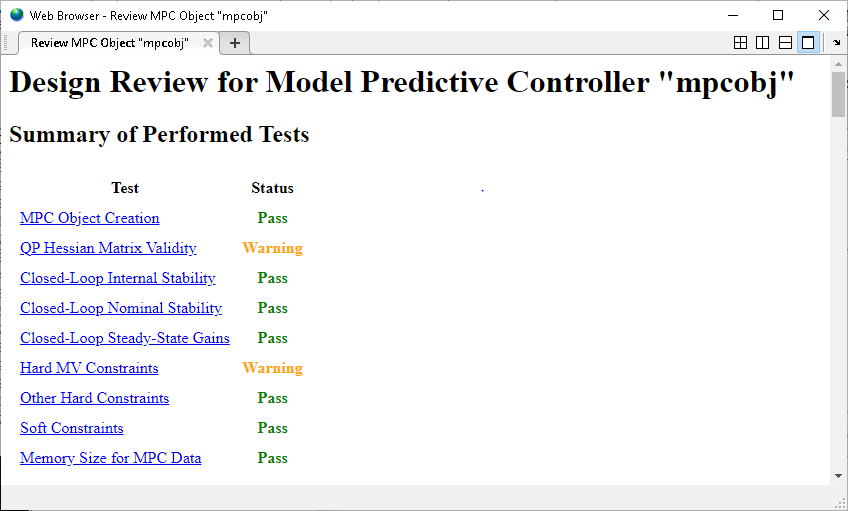In this example, the `review` command found two potential issues with the design. The first warning asks whether the user intends to have a weight of zero on the `C_A` output. The second warning advises the user to avoid having hard constraints on both `MV` and `MVRate`.

### Perform Linear Simulations

Use the `sim` function to run a linear simulation of the system. For example, simulate the closed-loop response of `MPCobj` for `26` control intervals. Specify setpoints of `2` and `0` for the reactor temperature and the residual concentration respectively. The setpoint for the residual concentration is ignored because the tuning weight for the second output is zero.

```T = 26; r = [0 0; 2 0]; sim(MPCobj,T,r) ```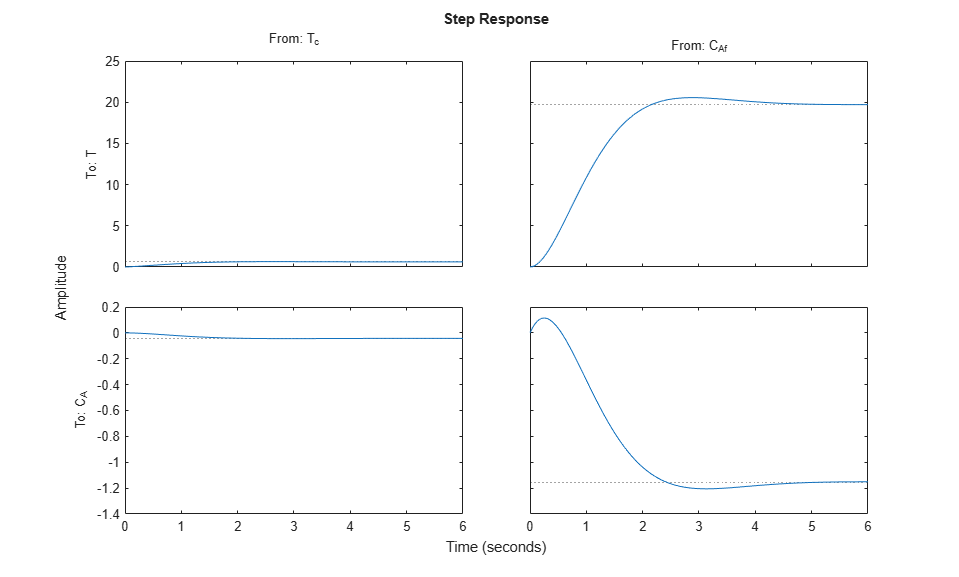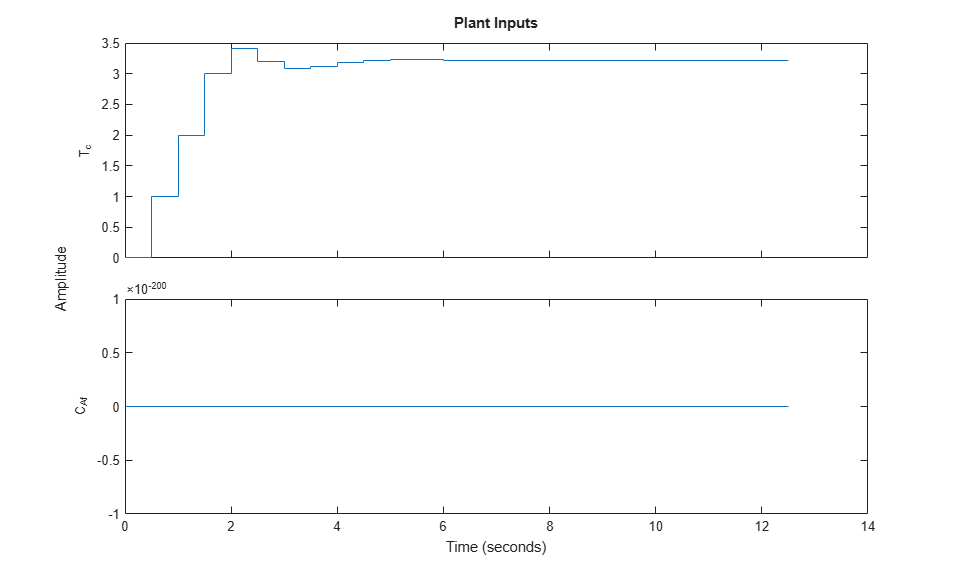You can modify the simulation options using `mpcsimopt`. For example, run a simulation with the manipulated variable constraints turned off.

```MPCopts = mpcsimopt; MPCopts.Constraints = 'off'; sim(MPCobj,T,r,MPCopts) ```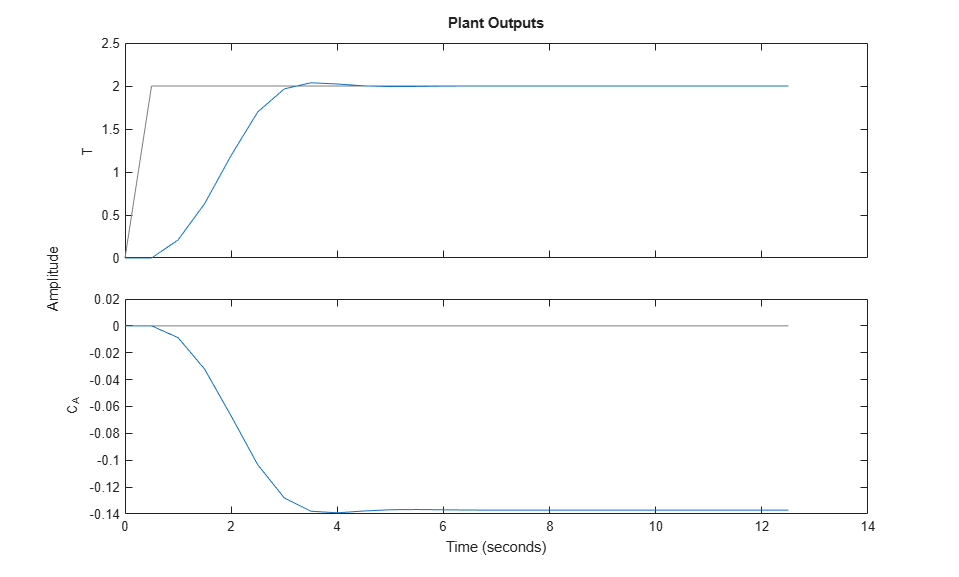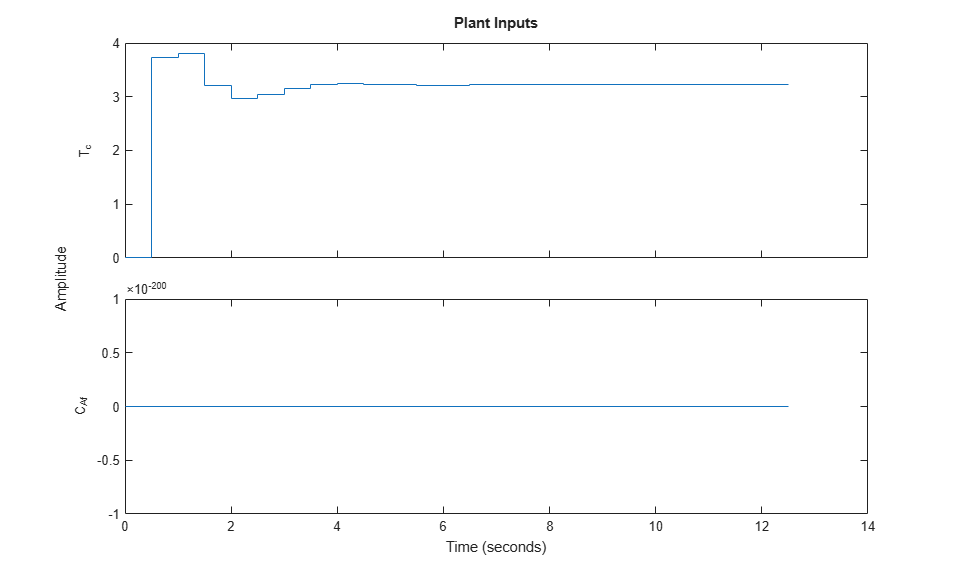The first move of the manipulated variable now exceeds the specified `3`-unit rate constraint.

You can also perform a simulation with a plant/model mismatch. For example, define a plant with 50% larger gains than those in the model used by the controller.

```Plant = 1.5*CSTR; MPCopts.Model = Plant; sim(MPCobj,T,r,MPCopts) ```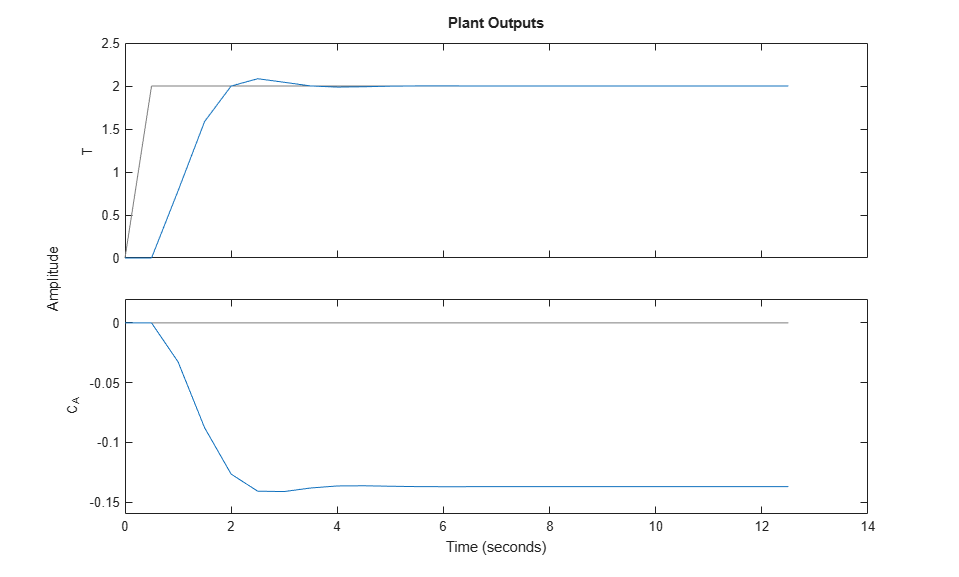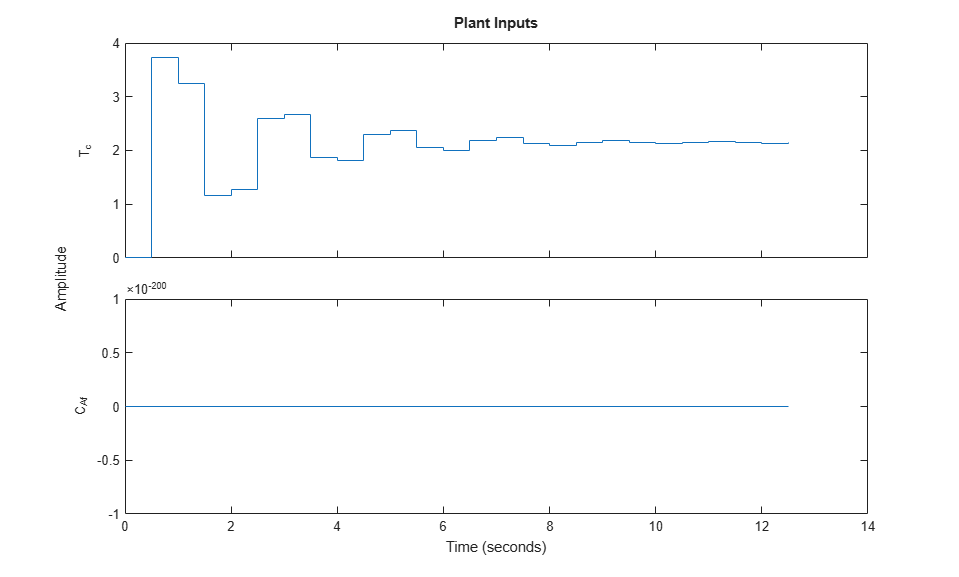The plant/model mismatch degrades controller performance slightly. Degradation can be severe and must be tested on a case-by-case basis.

Other options include the addition of a specified noise sequence to the manipulated variables or measured outputs, open-loop simulations, and a look-ahead option for better setpoint tracking or measured disturbance rejection.

### Store Simulation Results

Store the simulation results in the MATLAB Workspace.

```[y,t,u] = sim(MPCobj,T,r); ```

The syntax suppresses automatic plotting and returns the simulation results. You can use the results for other tasks, including custom plotting. For example, plot the manipulated variable and both output variables in the same figure.

```figure subplot(2,1,1) plot(t,u) title('Inputs') legend('T_c') subplot(2,1,2) plot(t,y) title('Outputs') legend('T','C_A') xlabel('Time') ```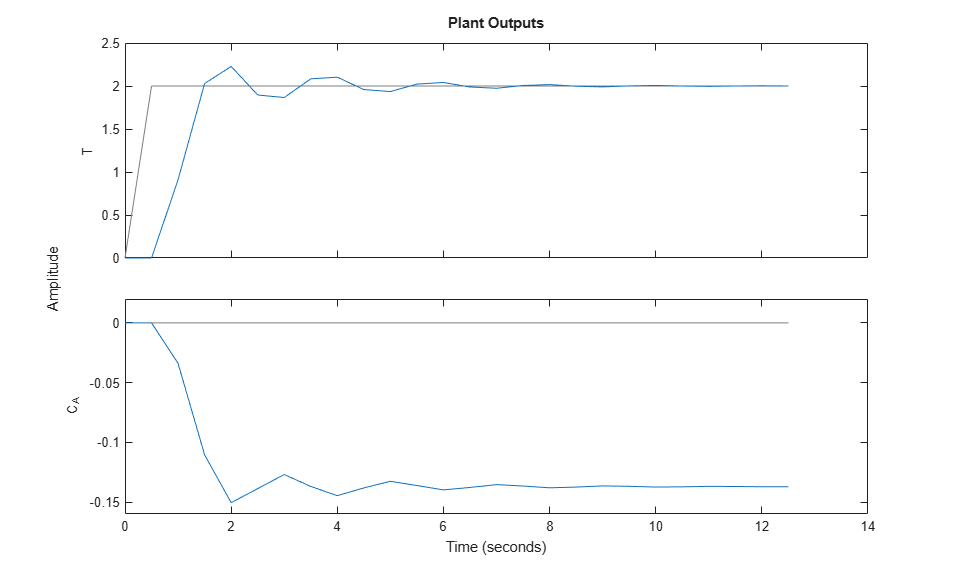Restore the `mpcverbosity` setting.

```mpcverbosity(old_status); ```